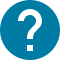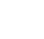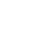学部後期課程
HOME 学部後期課程 最適化・意思決定論

# 最適化・意思決定論

Mathematical optimization in Operations Research
Operations Research (O.R.) is the application of scientific and mathematical methods to the study and analysis of problems involving complex systems (according to The Institute for Operations Research and the Management Sciences). Among many mathematical methods and tools in O.R., the most fundamental is the concept of optimization. It is to search for the best choice among possible alternatives under certain conditions and constraints. In the mathematical language, it is to maximize or minimize a given objective function by controlling decision variables under the set of constraints. Optimization theory is also a part of applied mathematics, and is widely employed in academic disciplines that deal with mathematical modeling of human decision making. In particular, it plays an essential role in economics for its descriptions of economic decision making and valuation.
In this lecture, students will learn the theories of mathematical optimization as methods in O.R. The lecture starts with providing an overview of O.R. with an emphasis on optimization methods. It moves on to the theory of static optimization. The third part of the lecture discusses the theory of dynamic optimization.

コース名

08D1426
FAS-DA4F26L3

A1 A2

2

NO

1. Introduction to O.R. and optimization Part I: Linear programing 2. The simplex method 3. Sensitivity analysis Part II: Nonlinear optimization theory 4. The Lagrange method 5. The Karush-Kuhn-Tucker (KKT) conditions 6. Duality 7. The envelope theorem 8. Multi-objective optimization Part III: Dynamic optimization 9. Calculus of variations 10. The maximum principle 11. Dynamic programming 12. Dynamical systems 13. Stochastic dynamic optimization

Lectures

Evaluation is based on take-home final exam (assignment). There will be no online exam. The details will be announced in due course.

No textbook. Handouts are available on ITC-LMS.

Recommended readings: Hillier, F. S. and G. J. Lieberman. Introduction to Operations Research, 10th Edition. McGraw Hill Higher Education, 2014. Intriligator, M.D. Mathematical Optimization and Economic Theory. Society for Industrial and Applied Mathematics, 2002. Sundaram, R.K. A First Course in Optimization Theory. Cambridge University Press, 1996.

Junior-Division math courses or similar level of calculus and linear algebra are prerequisite.